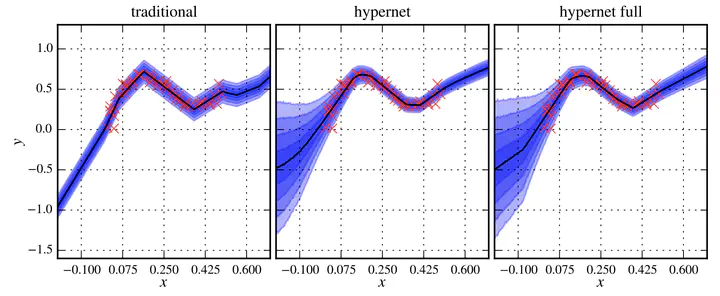ServiceNow Research

# Bayesian Hypernetworks## Abstract

We study Bayesian hypernetworks: a framework for approximate Bayesian inference in neural networks. A Bayesian hypernetwork $\h$ is a neural network which learns to transform a simple noise distribution, $p(\vec\epsilon) = \N(\vec 0,\mat I)$, to a distribution $q(\pp) := q(h(\vec\epsilon))$ over the parameters $\pp$ of another neural network (the “primary network”)@. We train q with variational inference, using an invertible $\h$ to enable efficient estimation of the variational lower bound on the posterior $p(\pp | \D)$ via sampling. In contrast to most methods for Bayesian deep learning, Bayesian hypernets can represent a complex multimodal approximate posterior with correlations between parameters, while enabling cheap iid sampling of~$q(\pp)$. In practice, Bayesian hypernets can provide a better defense against adversarial examples than dropout, and also exhibit competitive performance on a suite of tasks which evaluate model uncertainty, including regularization, active learning, and anomaly detection.

Type
Publication
Workshop at the Neural Information Processing Systems (NeurIPS)##### Alexandre Lacoste
###### Research Scientist

Research Scientist at Human Decision Support located at Montreal, QC, Canada.